GeeksforGeeks App
Open AppBrowser
Continue

# Conversion of Epsilon-NFA to NFA

Non-deterministic Finite Automata (NFA) is a finite automata having zero, one or more than one moves from a given state on a given input symbol. Epsilon NFA is the NFA which contains epsilon move(s)/Null move(s). To remove the epsilon move/Null move from epsilon-NFA and to convert it into NFA, we follow the steps mentioned below.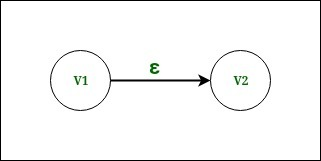Figure – Vertex v1 and Vertex v2 having an epsilon move

Step-1: Consider the two vertexes having the epsilon move. Here in Fig.1 we have vertex v1 and vertex v2 having epsilon move from v1 to v2.

Step-2: Now find all the moves to any other vertex that start from vertex v2 (other than the epsilon move that is considering). After finding the moves, duplicate all the moves that start from vertex v2, with the same input to start from vertex v1 and remove the epsilon move from vertex v1 to vertex v2.

Step-3: See that if the vertex v1 is a start state or not. If vertex v1 is a start state, then we will also make vertex v2 as a start state. If vertex v1 is not a start state, then there will not be any change.

Step-4: See that if the vertex v2 is a final state or not. If vertex v2 is a final state, then we will also make vertex v1 as a final state. If vertex v2 is not a final state, then there will not be any change. Repeat the steps(from step 1 to step 4) until all the epsilon moves are removed from the NFA. Now, to explain this conversion, let us take an example.

Example: Convert epsilon-NFA to NFA. Consider the example having states q0, q1, q2, q3, and q4.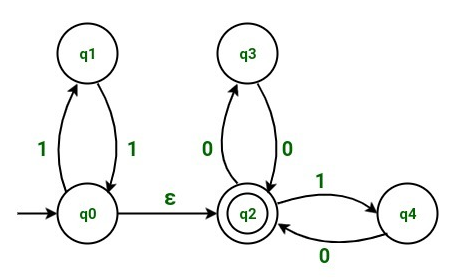In the above example, we have 5 states named as q0, q1, q2, q3 and q4. Initially, we have q0 as start state and q2 as final state. We have q1, q3 and q4 as intermediate states.

Transition table for the above NFA is:

According to the transition table above,

• state q0 on getting input 1 goes to state q1.
• State q0 on getting input as a null move (i.e. an epsilon move) goes to state q2.
• State q1 on getting input 1 goes to state q0.
• Similarly, state q2 on getting input 0 goes to state q3, state q2 on getting input 1 goes to state q4.
• Similarly, state q3 on getting input 0 goes to state q2.
• Similarly, state q4 on getting input 0 goes to state q2.

We can see that we have an epsilon move from state q0 to state q2, which is to be removed. To remove epsilon move from state q0 to state q1, we will follow the steps mentioned below.

Step-1: Considering the epsilon move from state q0 to state q2. Consider the state q0 as vertex v1 and state q2 as vertex v2.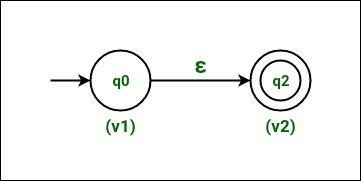Figure – State q0 as vertex v1 and state q2 as vertex v2

Step-2: Now find all the moves that starts from vertex v2 (i.e. state q2). After finding the moves, duplicate all the moves that start from vertex v2 (i.e state q2) with the same input to start from vertex v1 (i.e. state q0) and remove the epsilon move from vertex v1 (i.e. state q0) to vertex v2 (i.e. state q2). Since state q2 on getting input 0 goes to state q3. Hence on duplicating the move, we will have state q0 on getting input 0 also to go to state q3.

Similarly state q2 on getting input 1 goes to state q4. Hence on duplicating the move, we will have state q0 on getting input 1 also to go to state q4.

So, NFA after duplicating the moves is: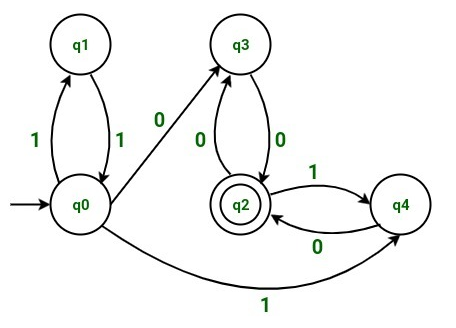Figure – NFA on duplicating moves

Step-3: Since vertex v1 (i.e. state q0) is a start state. Hence we will also make vertex v2 (i.e. state q2) as a start state. Note that state q2 will also remain as a final state as we had initially. NFA after making state q2 also as a start state is: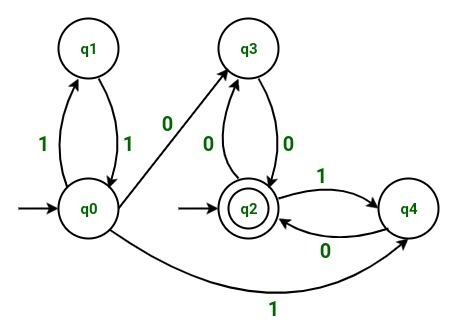Figure – NFA after making state q2 as a start state

Step-4: Since vertex v2 (i.e. state q2) is a final state. Hence we will also make vertex v1 (i.e. state q0) as a final state. Note that state q0 will also remain as a start state as we had initially. After making state q0 also as a final state, the resulting NFA is: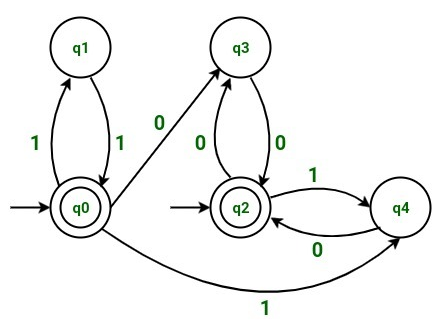Figure – Resulting NFA (state q0 as a final state)The transition table for the above resulting NFA is:

My Personal Notes arrow_drop_up